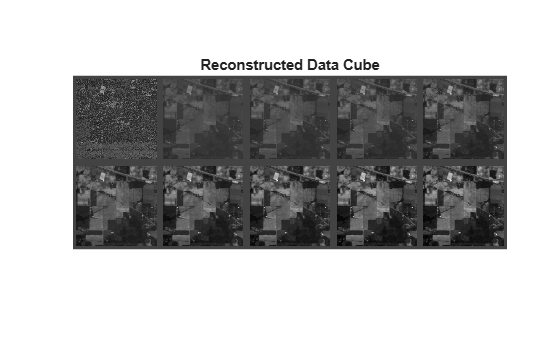# inverseProjection

Reconstruct data cube from principal component bands

Since R2020a

## Syntax

``reconstructedData = inverseProjection(pcDataCube,coeff)``

## Description

example

````reconstructedData = inverseProjection(pcDataCube,coeff)` reconstructs the original spectral bands in a hyperspectral data cube from the PCA (principal component analysis) or MNF (maximum noise fraction) transformed data cube and their related coefficients. NoteThis function requires the Image Processing Toolbox™ Hyperspectral Imaging Library. You can install the Image Processing Toolbox Hyperspectral Imaging Library from Add-On Explorer. For more information about installing add-ons, see Get and Manage Add-Ons.The Image Processing Toolbox Hyperspectral Imaging Library requires desktop MATLAB®, as MATLAB Online™ or MATLAB Mobile™ do not support the library. ```

## Examples

collapse all

Read a hyperspectral data into the workspace.

`hcube = hypercube('indian_pines.dat');`

Extract 10 principal component bands and the transformation coefficients using the principal component analysis method.

`[pcDataCube,coeff] = hyperpca(hcube,10);`

Reconstruct the original data from 10 principal component bands.

`reconstructedData = inverseProjection(pcDataCube,coeff);`

Display the first 10 spectral bands in input data cube.

```figure montage(hcube.DataCube(:,:,1:10),'BorderSize',[10 10],'Size',[2 5],'DisplayRange',[]); title('Original Data Cube')```Display the first 10 spectral bands in the reconstructed data cube.

```figure montage(reconstructedData(:,:,1:10),'BorderSize',[10 10],'Size',[2 5],'DisplayRange',[]); title('Reconstructed Data Cube')```## Input Arguments

collapse all

PCA or MNF transformed data cube, specified as a 3-D numeric array of size M-by-N-by-P. The PCA or MNF transformed data cube of a hyperspectral data cube is computed using the `hyperpca` or `hypermnf` functions respectively. P specifies the number of principal component bands in the transformed data cube.

Data Types: `single` | `double` | `int8` | `int16` | `int32` | `int64` | `uint8` | `uint16` | `uint32` | `uint64`

PCA or MNF coefficients, specified as a matrix of size C-by-P.

Data Types: `single` | `double` | `int8` | `int16` | `int32` | `int64` | `uint8` | `uint16` | `uint32` | `uint64`

## Output Arguments

collapse all

Reconstructed data cube, returned as a 3-D numeric array of size M-N-by-C. The data type of the reconstructed data cube is same as that of the transformed data cube at the input.

Data Types: `single` | `double` | `int8` | `int16` | `int32` | `int64` | `uint8` | `uint16` | `uint32` | `uint64`

## Version History

Introduced in R2020a